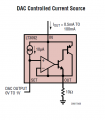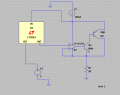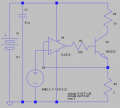# How can i make a power supply with arduino due?

#### tuto

Joined May 2, 2021
15
hello everyone, i got a question:

I need to make a power supply to heat a heater, for that I need to use an arduino due, and use one of the analog pins (it´s like a built-in DAC) of this microcontroller, and draw voltage from that pin, with an external current source or something similar, I need power control the current that passes through the circuit controlled from arduino, controlling the voltage that I give it through the software, that is, I choose the voltage that I pass to the circuit with the arduino's analog pin and with this choice, I have to have a certain current that allows me to heat the heater resistance as much as I want. I need to give the resistor a power of 10W, and the resistance of the heater is 50Ω. so I need a current of approximately 400mA to pass through that resistance. How can I do this circuit? the main problem I've had so far is that the output current is always very small. any suggestions? thnks.

#### wayneh

Joined Sep 9, 2010
17,466
So do you want to control a temperature (which will require a sensor) or the current through the heater element? And is 400mA the maximum current you may need?

You need to put a power transistor under the control of your Arduino, and that will control the heater current.

•anniel747

#### MrChips

Joined Oct 2, 2009
29,809
Power is calculated knowing two of three things: V, I , R.

Using Ohm's Law, you can substitute:
I = V / R
V = I x R
R = V / I

Power is:
P = V x I
P = V x V / R
P = I x I x R

For 10W into 50Ω you will need 24V supply.
Arduino circuitry cannot supply 24V. You can use Arduino to switch 24V supply on and off.
Use PWM to control the 24V supply and hence control the power to the 50Ω load.

•anniel747

#### BobTPH

Joined Jun 5, 2013
8,075
What is the Arduino doing other than putting out the control voltage? Sounds like you are using the Arduino as a potentiometer.

Bob

•anniel747

#### wayneh

Joined Sep 9, 2010
17,466
I took for granted but neglected to mention that you need a source of power external to the Arduino. @MrChips is correct. You haven't mentioned what power source you have available.

Arduino circuitry cannot supply 24V. You can use Arduino to switch 24V supply on and off.

•anniel747

#### Papabravo

Joined Feb 24, 2006
20,597
I'm not at all clear on the purpose of the Arduino. Temperature control systems seldom require continuous control and monitoring. Fast response times are completely wasted.

•anniel747

#### Irving

Joined Jan 30, 2016
3,536
Sounds like a homework problem.

You don't need an Arduino, it can be done with purely analog circuitry.

However, if you must use an Arduino you need (as already said):
a) A suitable power supply of >= 24v
b) An output stage to control the current in the heater.
(i)This could be analog/linear where the Arduino outputs a voltage to control the heater current and varies this according to the temperature measured using the Arduino's analog input; or
(ii) Digital, where the Arduino switches the heater current on and off quickly (eg 100 - 10000 times/sec). The ratio of on to off , the duty cycle, generates an average current in the heater. The Arduino varies the duty cycle according to the measured temperature. This is the preferred approach as it is far more efficient.
c) A control algorithm for the Arduino to relate output to input, in some proportional manner, the so-called PID controller.

•anniel747

#### tuto

Joined May 2, 2021
15
I was trying to do the following. now being more explicit. I want to use the dac, to be choosing the voltage each time and that the circuit gives me the current that I need to heat the 50Ω resistance(10KΩ in the image). I reproduced the circuit in the image in ltspice(i don´t have a dac on ltspice so i put a fixed voltage source) but the problem I have is that the current that enters on the circuit through my current generator practically does not vary at the output when changing the voltage with the DAC. if i put 100mA , approximately 100 mA pass through the output (50 ohms resistor) no matter what voltage value I put on the voltage source (or in my dac) .(of course the current is always fixed ).what's wrong with that circuit?#### Irving

Joined Jan 30, 2016
3,536
Without trying it myself my guess is that the opamp simulation isn't working. You don't have a voltage source in your circuit. Replace the current source with a voltage source of 24v and I think you'll find it works. The opamp /transistor pair control the output current but with just a current source theres never going to be a sufficient potential difference to allow them to take control. Think of your Kirchhoff current law - treating that as a black box there's only one way for current to go in and one out via the resistor so the current through the resistor must be the same as the current source.

Also for the simulation to be useful you need to choose representative transistors rather than generic NPN & PNP..

•tuto

#### wayneh

Joined Sep 9, 2010
17,466
For the record you need only an op-amp to accomplish your goal, along with a power transistor.

You use a low ohms shunt resistor (eg. 2Ω) to convert the the heater current to a voltage (eg. 0.5A x 2Ω = 1V). Compare the shunt voltage to the 'reference' voltage input, V2. The opamp output drives a transistor to control the heater current. Here's an example. R3 is your heater and R4 is the shunt.There are many similar ways to set this up. For instance you could use the heater as the shunt and amplify the input voltage to match the voltage required across the heater. Point is, there are many ways to accomplish this and adding a micro really doesn't help much.

•tuto, anniel747 and Irving

#### Irving

Joined Jan 30, 2016
3,536
Going back to the TS original post, his heater is 50ohm @ ~450mA for 10W output. That needs 50 * 0.45 = 22.5v across the heater, so at least a 24v supply. That leaves 1.5v across the transistor and current sense resistor. At 2ohm the latter dissipates 0.4W and drops 0.9v leaving 0.6v across the transistor which will be close to saturation and dissipates 0.27W (for a junction temp of ~80C and a case temp of ~57C, just about OK for a 2N2222). Personally I'd prefer to see a higher voltage, or a smaller heater resistor/higher current to give more room to move. I'd use a smaller feedback resistor, say 1ohm and a second opamp to amplify the sense voltage from 0.45v to around 2v (G= 4 or 5). A higher current will need a beefier transistor, or better, a MOSFET.

•tuto and anniel747

#### wayneh

Joined Sep 9, 2010
17,466
All true. I was just giving an example without spending the time to optimize it. Too many unknowns for that.